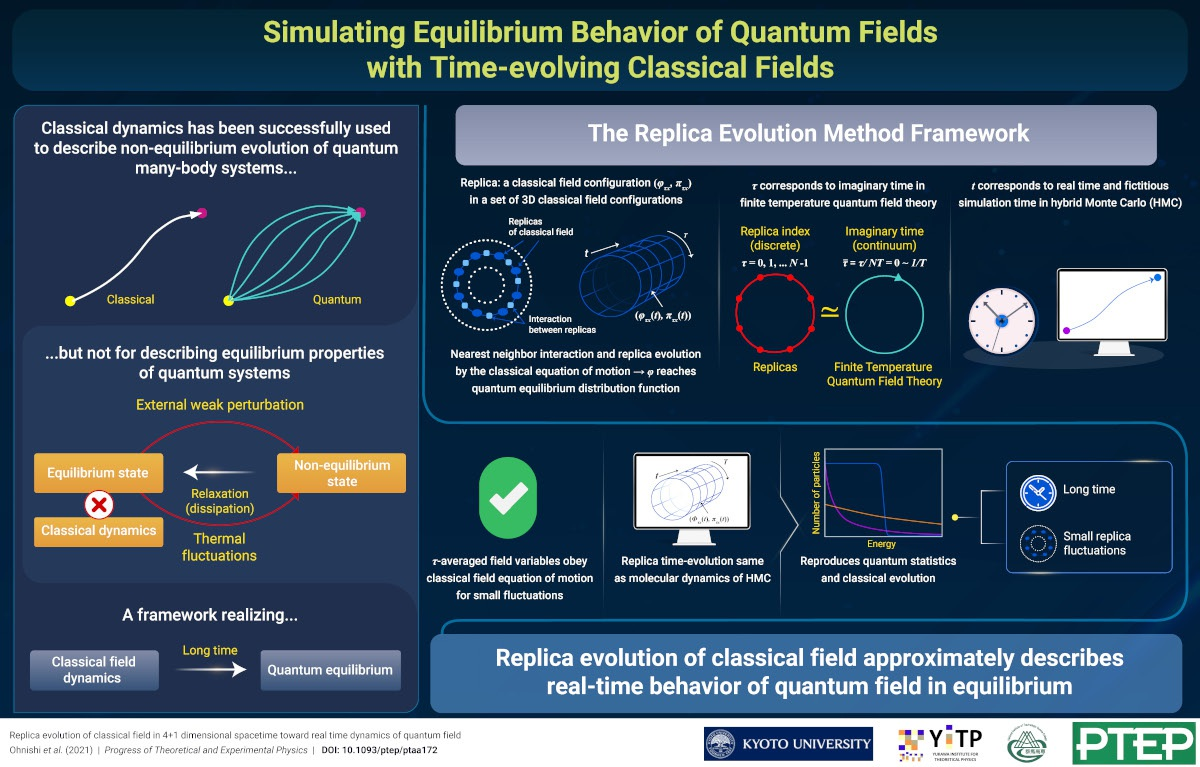# Simulating Equilibrium Behavior of Quantum Fields with Time-evolving Classical Fields

2021-4-5

JPS Hot Topics 1, 031

https://doi.org/10.7566/JPSHT.1.031

© The Physical Society of Japan

Replica evolution of classical field in 4+1 dimensional spacetime toward real time dynamics of quantum field
(PTEP Editors' Choice)

Akira Ohnishi, Hidefumi Matsuda, Teiji Kunihiro, and Toru T. Takahashi
Prog. Theor. Exp. Phys. 2021, 023B09 (2021).

A set of field configurations (replicas) reaches equilibrium of quantum field theory after real-time evolution obeying classical equations of motion.While classical and quantum regimes are typically regarded as distinct, classical dynamics can, in fact, be used to determine the time evolution of a quantum many-body system under non-equilibrium, thus doing away with a full-blown quantum machinery. Essentially, the classical equation of motion for the phase space distribution can provide an approximate solution of the quantum equation of motion for the density matrix (matrix describing a quantum system’s statistical state), if the initial classical analogue of the quantum distribution function is known. This beneficial feature of classical dynamics can even be extended to the case of field theories, where, instead of discrete degrees of freedom, we deal with continuous degrees of freedom.

However, there is a caveat: the classical dynamics approach is less useful when describing equilibrium behavior of quantum systems. For instance, in the case of black-body cavity radiation, classical considerations lead to the Rayleigh-Jeans divergence. Thus, a framework that can help us reach quantum statistical equilibrium following a classical dynamical evolution is highly desired.

To that end, the authors propose a novel framework called “the replica evolution method” in which quantum statistics is realized by solving classical equations of motion. To start with, they consider a set of classical field configurations (Φ) in (3+1) dimensions (three spatial dimensions, one replica index (τ) dimension), called “replicas,” where each replica interacts with its nearest neighbors through a τ-derivative term that eventually leads to their thermalization. The time evolution of the replicas is governed by the classical Hamilton’s equation of motion.

The most interesting feature of their framework is that the replica index ‘τ’ can be regarded as the imaginary time index in finite temperature quantum field theory, so that the replica evolution is technically similar to the fictitious time evolution in a hybrid Monte Carlo simulation. This, in turn, implies that, given a distribution of initial replica Hamiltonian values, the replicas should relax to the equilibrium quantum distributions following a long-time evolution. Moreover, the τ-averaged field variables obey classical equations of motion when the fluctuations among replicas are small. From these two aspects, one can expect that replica evolution would describe the real time evolution of quantum systems. Actually, the time-correlation function in the replica evolution is found to reproduce that in quantum field theory to a good approximation. This framework can thus allow for more precise predictions of relaxation processes in quantum systems and possibly help unify the background field and the particle degrees of freedom, paving the way for an integrated transport theory.

Replica evolution of classical field in 4+1 dimensional spacetime toward real time dynamics of quantum field
(PTEP Editors' Choice)

Akira Ohnishi, Hidefumi Matsuda, Teiji Kunihiro, and Toru T. Takahashi
Prog. Theor. Exp. Phys. 2021, 023B09 (2021).